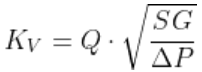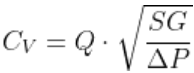# Flow Coefficient calculator, Cv or Kv, for liquids

## Calculator

Calculate Parameter Value unit
Flow Coefficient
( ):
Flow:
Specific Gravity:Pressure Drop:

## Theory

Metric Units:
The flow factor, or flow coefficient of a valve shows the relationship between the pressure drop and the flow rate. Kv is the flow coefficient in metric units, and can be calculated with the following equation:where
KV: Flow factor (m3·h-1·bar-0.5)
Q: Flow rate (m3/h)
SG: Specific Gravity of the liquid (dimensionless). For water SG=1
ΔP: Pressure drop - difference between inlet and outlet pressure - (bar)
Imperial units:
In imperial units the flow coefficient is called CV:where
CV: Flow coefficient
Q: Flow rate (US gallons per minute)
SG: Specific Gravity of the liquid (dimensionless). For water SG=1
ΔP: Pressure drop - difference between inlet and outlet pressure - (psi)